# NOTE: This set of www pages is not the set of www pages for the curent version of COMP101. The pages are from a previous version that, at the request of students, I have kept on line.

## CONTENTS

 1. Selection 2. "If-else" statements 3. Example problem - power 4 3.1. Requirements 3.2. Analysis 3.3. Design 3.4. Implementation 3.5. Testing ` ` 4. Black box and white box testing 5. Example problem - linear equations (includes an example of a Boolean function) 5.1. Requirements 5.2. Analysis 5.3. Design 5.4. Implementation 5.5. Testing 6. Summary

First example includes a simple if, and second example an "if-else". The second example also includes a cast (for revision purposes).

## 1. SELECTION

A selection statement provides for selection between alternatives. We can identify two broad types of selection construct:

1. If-else statements
2. Switch (or case) statements

## 2. "IF-ELSE" STATEMENTS

 An "if-else" statement, sometimes referred to as a conditional, can be used in two forms (the first is often referred to as a linear if): ```if ( < Condition X> ) { < Action Y> } ``` ```if ( < Condition X> ) { < Action Y> } else { < Action Z> } ``` Where the condition is a Boolean expression (or a sequence of Boolean expressions), and the action part comprises one or more statements. Note that where the action part comprises only a single statement we can omit the "curly" brackets. We can also identify a number of variations of the above which are particular to individual programming languages. For example in Java we can write: ` ` ```if < Condition X1> < Action Y1> ; else if < Condition X2> < Action Y2> ; ... else if < Condition Xn> < Action Yn> ; else < Action Z> ; ``` The following are all examples of valid Boolean expressions, or sequences there of, that may form the condition part of "if-else" statements: ```if (valueA < 50) ... ; if (valueB == 0) ... ; if (valueX > 10 & valueX < 20) ... ; if (valueX < 10 | valueX > 20) ... ; if (valueA <= 20.0 & (valueB != 'q' | testFun(valueA,valueC) == 1)) ... ; ```

## 3. EXAMPLE PROBLEM --- POWER 4

### 3.1 Requirements

Design and create a Java program that takes a single integer as input. If the input is less than 50 adds 10 and returns the result raised to the power of 4, otherwise simply returns the input raised to the power of 4 (Figure 1).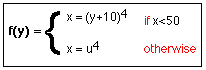Figure 1: Power 4 problem

### 3.2 Analysis

A class diagram for the proposed solution is given in Figure 2.

### 3.3 Design

From Figure 2 it can be seen that we require only one class, Power4App.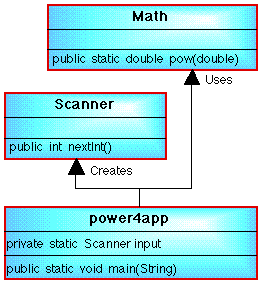Figure 2: Power 4 class diagram

#### 3.3.1 Power4app Class

 Field Summary private static Scanner input            A class instance to facilitate input from the input stream.

 Method Summary public static void main(String[] args)            Main (and only) method for resolution of problem. Takes input, carries out check on input using a simple if, and then outputs the result. A data table for the method is given to the right.

 A Nassi-shneiderman chart is given in Figure 3. Note the way that the "choice point" is incorporated into the chart. If the test is satisfied (i.e. produces an anonymous data item of type Boolean which has the value true then flow of control proceeds down the left hand side of the chart. However, if the result is false then flow of control proceeds down the right hand side.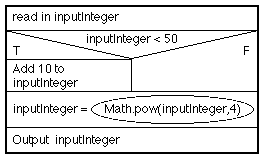Figure 3: Nassi-Shneiderman charts for Power4app class main method
 It is sometimes useful to produce an activity diagram (Figure 4) indicating flow of control through a method. Such a diagram is presented in Figure 4. The choice point (selection) is indicated by a "diamond", other sequences of statements by simple boxes. Flow of control is indicated by the directed lines. Flow starts at the "start" oval (referred to as the riser) and ends at the "end" oval (referred to as the sink). Thus we can imagine the flow of control being indicated by a fluid that comes out of the "riser", flows through the system, and disappears down the "sink". The principal advantages of such a diagram is that it clearly indicates the paths through the system, essential information required when testing software systems that include choice points (see below).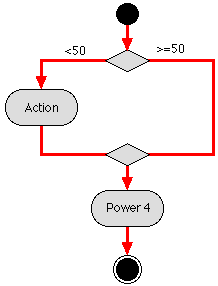Figure 4: Activity Diagram for Power4app class main method

### 3.3. Implementation

 ```// POWER 4 APPLICATION // Frans Coenen // Tuesday 22 March 1999 // Revised: Wednesday 5 July 2005 // The University of Liverpool, UK import java.util.*; class Power4app { // ------------------- FIELDS ------------------------ // Create Scanner class instance private static Scanner input = new Scanner(System.in); // ------------------ METHODS ------------------------ /* Main method */ public static void main(String[] args) { int inputInteger; // Input System.out.print("Input an integer: "); inputInteger = input.nextInt(); // Calculation if (inputInteger < 50) inputInteger += 10; inputInteger = (int) Math.pow((double)inputInteger,4.0); // Output System.out.println("Result is: " + inputInteger); } } ```

Table 1: Power 4 application class

### 3.4. Testing

1. Arithmetic testing: We should derive test cases incorporating positive, negative and zero sample values. An appropriate set of cases is given in the table to the right.

TEST CASEEXPECTED RESULT
inputIntegerOUTPUT
-10 0
0 10000
10160000

2. Path testing: An "if" statement should always be tested using at least two test cases, one that causes the if statement to succeed and one that causes it to fail. In other words both "paths" through the procedure should be tested. Consequently this type of testing is referred to as path testing. It is also a good idea, when using discrete data items in the condition expression, to test at the "cut-off" point where appropriate, i.e. 49 and 50 in this case (in the spirit of range and limit testing). A suitable set of test cases are given in the table to the right.

TEST CASEEXPECTED RESULT
inputIntegerOUTPUT
10 160000
4912117361
50 6250000
5912117361
Note that, as we would expect, inputs of 49 and 59 produce the same result. Note also that one of the test cases was also included in the arithmetic test case set. It is a good idea to "double-up" test cases where ever possible (i.e. design a test cases that address several aspect of the software at the same time), as this reduces the overall testing effort required.

3. Data validation: In addition we should undertake some data validation testing

## 4. BLACK BOX AND WHITE BOX TESTING

 Techniques such as arithmetic testing are generally regarded as Black Box Testing techniques. The term is intended to convey the idea that the software to be tested is to be regarded as a box, the internal workings of which cannot be seen, into which we feed input data as a result of which output data is produced. Thus black box test cases are only concerned with input and output and not the nature of the transformation(s) that causes the first to become the second. In some cases, for example arithmetic testing, we may make use of knowledge of the inner workings of a program to reduce the number of test cases that need to be considered, but such tests are still considered to be black box in nature. ` ` The opposite of black box testing is white box (or glass box) testing. White box testing is concerned with the inner workings of a software systems. Path testing, as demonstrated above, is an example of a while box testing technique. Data validation is not generally considered to fall into either the black box or white box categories.

## 6. "IF-ELSE" SUMMARY

Here we have described the selection process using an "if-else" statement. This comes in two forms:

1. Simple: "If" part of statement only, no "else" part.
2. Standard: Both "if" and "else" parts.

A generic Nassi-Shneiderman charts and Activity Diagram for both forms are given in Figure 9.

Simple (Lineaer) ifStandard if-else
Activity Diagrams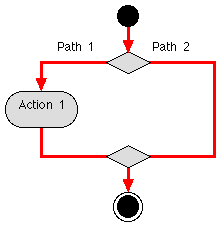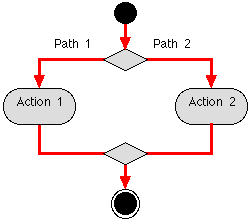Nassi-Shneiderman Charts>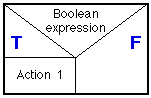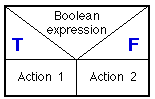Figure 9 Generic Activity Diagrams and Nassi-Shneiderman charts "if-else" statements (simple/linear and standard forms)

Created and maintained by Frans Coenen. Last updated 10 February 2015